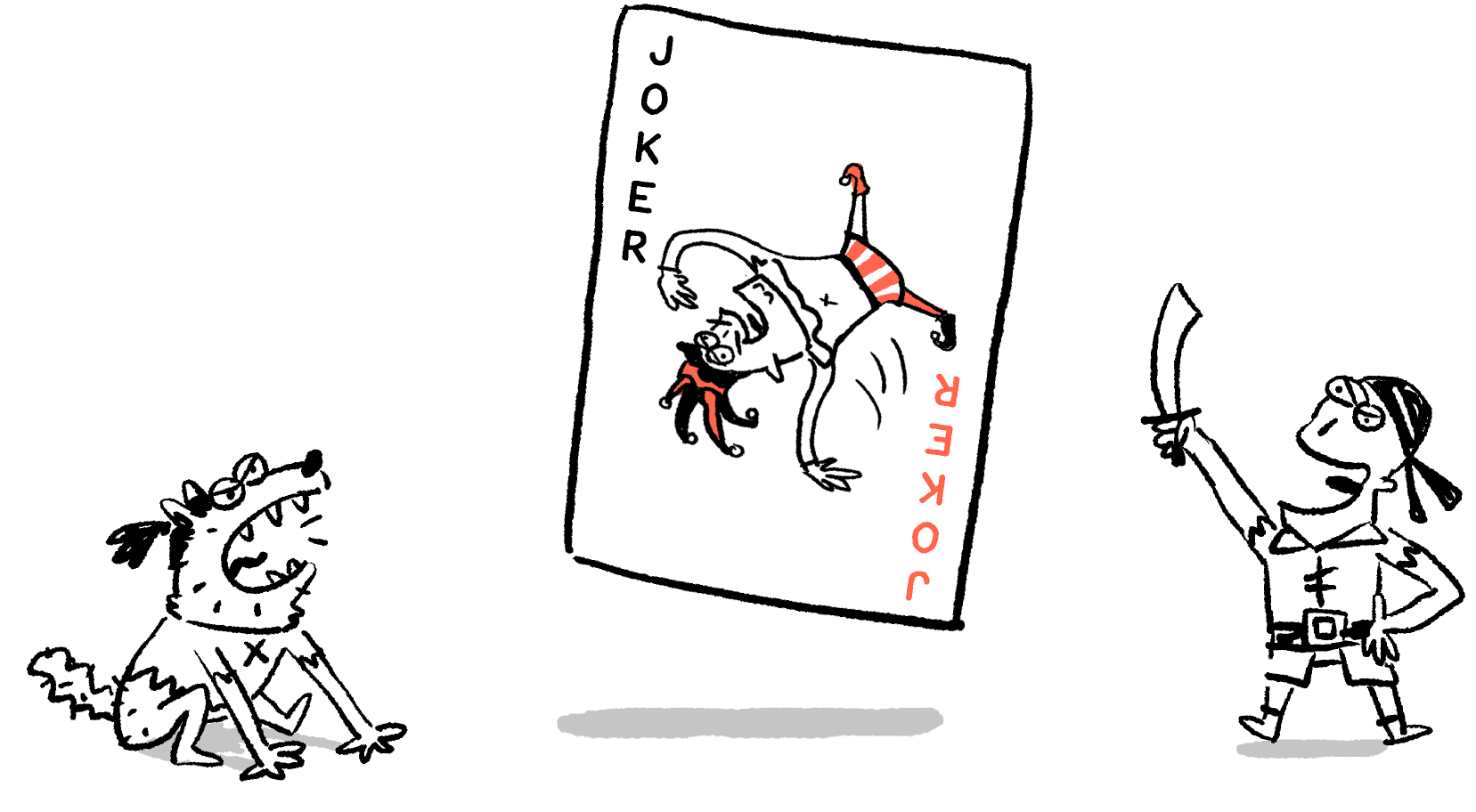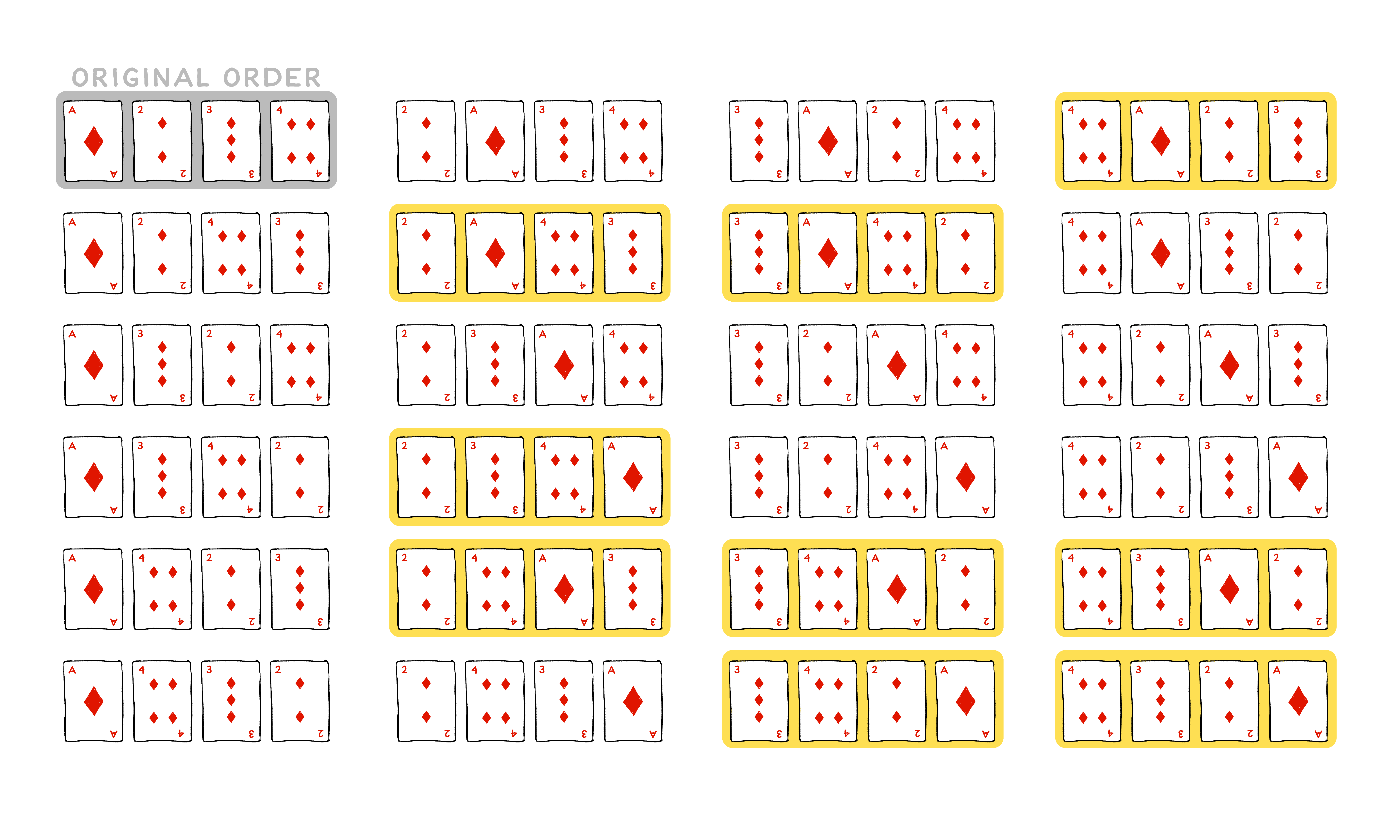Back

## BFF Queens

Even simple probability problems can defy our intuition. Although the basic idea of probability is straightforward, the details can be quite subtle.Keep reading for an example problem involving card arrangements, or jump straight to today's challenge.

To start, let's consider a scenario that's very similar to today's problem.

Suppose you have a four-card deck that starts in a specific order, and then you thoroughly shuffle it. In today's challenge, that's exactly what you have, and you're calculating the probability that, after the shuffle, the original second and third cards are still next to each other. In this explanation, we'll tackle a slightly different but related question: How many of the potential results of thoroughly shuffling of a four-card deck will have no cards that are still in their original positions? This is called a "derangement" of the deck.

First, let's consider all of the different ways that you can order four unique cards. To make it easy to talk about the order, we'll use the ace, two, three, and four of diamonds as our four-card deck. The chart below shows all $24$ of the ways these four cards can be arranged after a shuffle, including the original order: ace-two-three-four. If the shuffle is thorough, each of these $24$ arrangements will be equally likely.But how do we know that this is truly all of the possible arrangements? Think about it like this: if you're trying to make an arrangement of these four unique cards, you get to make three choices:

1. You can choose any one of the four cards to put as the first card.
2. Next, you can choose any of the three remaining cards to put as the second card.
3. Last choice: pick either of the two remaining cards to put third.

Finally, you're holding just one card, and you must put that card at the end.

If you look at the chart above, the possibilities are grouped based on these four choices. For each of the four possible first card, there are six different arrangements, with two of each of the three remaining cards in the second position. These $24$ different arrangements are called the $24$ possible permutations of the four-card deck.

Now that we're certain that these are all of the possible orderings, we can just examine them all and count the derangements. If you try it yourself, you should be able to find $9$ derangements, aka, $9$ arrangements where none of the cards are in their original positions. For the other $24-9=15$ arrangements, at least one of the four cards is in the same position as it was in originally.

Lastly, let's phrase this as a probabilistic result. Since each of the $24$ orders is equally likely, after the shuffle, there's a $\frac{9}{24} = \frac{3}{8}$ probability that, after a shuffle, none of the four cards would be in their original positions.

# Today's Challenge

If you shuffle the four cards below and then deal them out in a row on a table, what is the probability that the two queens are dealt out side by side?×